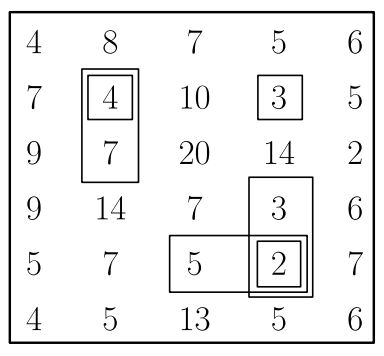시간 제한메모리 제한제출정답맞힌 사람정답 비율
5 초 1024 MB176302822.581%

## 문제

In the early 19th century, the ruler Hoseyngulu Khan Sardar ordered a palace to be built on a plateau overseeing a beautiful river. The plateau is modeled as an $n \times m$ grid of square cells. The rows of the grid are numbered $0$ through $n-1$, and the columns are numbered $0$ through $m-1$. We refer to the cell in row $i$ and column $j$ ($0 \leq i \leq n-1, 0 \leq j \leq m-1$) as cell $(i,j)$. Each cell $(i,j)$ has a specific height, denoted by $a[i][j]$.

Hoseyngulu Khan Sardar asked his architects to choose a rectangular area to build the palace. The area should not contain any cell from the grid boundaries (row $0$, row $n-1$, column $0$, and column $m-1$). Hence, the architects should choose four integers $r_1$, $r_2$, $c_1$, and $c_2$ ($1 \leq r_1 \leq r_2 \leq n-2$ and $1 \leq c_1 \leq c_2 \leq m-2$), which define an area consisting of all cells $(i, j)$ such that $r_1 \leq i \leq r_2$ and $c_1 \leq j \leq c_2$.

In addition, an area is considered valid, if and only if for every cell $(i, j)$ in the area, the following condition holds:

• Consider the two cells adjacent to the area in row $i$ (cell $(i, c_1-1)$ and cell $(i, c_2+1)$) and the two cells adjacent to the area in column $j$ (cell $(r_1-1, j)$ and cell $(r_2+1, j)$).

The height of cell $(i,j)$ should be strictly smaller than the heights of all these four cells.

Your task is to help the architects find the number of valid areas for the palace (i.e., the number of choices of $r_1$, $r_2$, $c_1$ and $c_2$ that define a valid area).

## 구현

You should implement the following procedure:

int64 count_rectangles(int[][] a)

• $a$: a two-dimensional $n$ by $m$ array of integers representing the heights of the cells.
• This procedure should return the number of valid areas for the palace.

## 제한

• $1 \leq n, m \leq 2500$
• $0 \leq a[i][j] \leq 7\,000\,000$ (for all $0 \leq i \leq n-1, 0 \leq j \leq m-1$)

## 예시 1

Consider the following call.

count_rectangles([[4,  8,  7,  5,  6],
[7,  4,  10, 3,  5],
[9,  7,  20, 14, 2],
[9,  14, 7,  3,  6],
[5,  7,  5,  2,  7],
[4,  5,  13, 5,  6]])There are $6$ valid areas, listed below:

• $r_1 = r_2 = c_1 = c_2 = 1$
• $r_1 = 1, r_2 = 2, c_1 = c_2 = 1$
• $r_1 = r_2 = 1, c_1 = c_2 = 3$
• $r_1 = r_2 = 4, c_1 = 2, c_2 = 3$
• $r_1 = r_2 = 4, c_1 = c_2 = 3$
• $r_1 = 3, r_2 = 4, c_1 = c_2 = 3$

For example $r_1 = 1, r_2 = 2, c_1 = c_2 = 1$ is a valid area because both following conditions hold:

• $a=4$ is strictly smaller than $a=8$, $a=14$, $a=7$, and $a=10$.
• $a=7$ is strictly smaller than $a=8$, $a=14$, $a=9$, and $a=20$.

## 서브태스크

번호배점제한
18

$n, m \leq 30$

27

$n, m \leq 80$

312

$n, m \leq 200$

422

$n, m \leq 700$

510

$n \leq 3$

613

$0 \leq a[i][j] \leq 1$ (for all $0 \leq i \leq n-1, 0 \leq j \leq m-1$)

728

## 출처• 문제를 만든 사람: Peyman Jabbarzade

## 제출할 수 있는 언어

C++17, C++14, C++20, C++14 (Clang), C++17 (Clang), C++20 (Clang)

## 채점 및 기타 정보

• 예제는 채점하지 않는다.